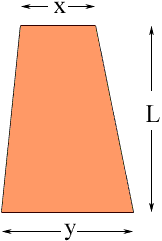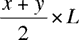Quandaries and Queries My husband works with asphalt building roads.  There are times when one end of the road will be (for example) 100ft wide the other end would be( for example) 200ft wide and he must figure the area in square feet.  So far it has been a guessing game because he dosen't have the formula to figure the square feet.  HELP!!! Hi, Start by looking at one end of the trapezoid.Take a point on the long side that is half way between the end of the short side and the end of the long side and construct a rectangleImagine that you construct an asphalt rectangle of this size.If you could just cut off the red triangular corner in the diagram below and move it down to cover the green triangle you would have exactly what you need.Of course you can't do this but it does show you how to find the area of a trapezoid. Find a quantity that is half way between the length of the long side and the length of the short side, and multiply by the height of the trapezoid. To be more explicit, in the diagram below,x is the length of the short side in feet, y is the length of the long side in feet and L is the height of the trapezoid in feet, then the area in square feet isCheers, Penny Go to Math Central